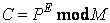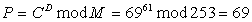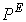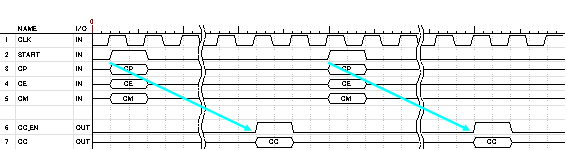Lib4U

‎"Behind every stack of books there is a flood of knowledge."

RSA Public Key Encryption System1．Introduction

This year’s design target is a RSA public key encryption CODEC. You may choose either Level 1 or Level 2 design specification depending on your skills. Enjoy RTL design and let us meet together at beautiful coral island Okinawa.

2．Basics

2.1 Some definition

Public key encryption algorithm has been developed by Rivest, Shamir, and Adleman in MIT as pioneers work. By taking their initial name, this algorithm is called RSA-encryption system. The RSA encryption algorithm is widely used such as for emails and files encryption system called PGP(Pretty Good Privacy) , for e-commerce encryption system called SSL(Secure Socket Layer) and so on.
In spite of widely used encryption algorithm, the RSA requires a lot of time for encryption and decryption of plane text. This is due to its heavy computational complexity since it makes use of prime factorization with respect to two prime number product.
This year’s design target is to design an encryption or a decryption system with fewer computations(high-speed) and/or less number of gates as much as possible.Fig. 1  RSA Encryption system

2.2 RSA Encryption Algorithm

Let us define some integer parameters P as a plane text, C as an encrypted text, E as the encryption key, D as the decryption key, and M as modulo number. The encryption can be made by following equation.(1)

where mod expresses a modular operation.

On the other hand, the following equation is used for decryption.(2)

As we can see in Eq.(1) and (2), the RSA encryption system’s expression itself is not complicated.
At the first step how to define E, D and M. we randomly choose quite large number of two prime factors p and q (p≠q). Then modulo M is defined as M=p×q. From p and q, we define(3)

where LCM stands for the least common multiple.
The encryption key is chosen as a certain number which is less than and mutually prime with L. Therefore E can be expressed as(4)

where GCD stands for the greatest common devisor.
In order to shorten the processing time(computational complexity), E is often chosen to be relatively small number.
Lastly, the decryption key D should satisfy the following equation for arbitrary integer number H.(5)

By using above defined E, D and M, encryption and decryption can be carried out according to the Eq.(1) and (2). See some references for more theoretical explanation.
As you might already realize it, the decryption key D can be easily obtained if the modulo M can be prime factorized; however, as far as M is chosen as several hundred digits, prime factorization would take more than several to 10 years or more even by using a state-of-the-art super computers. This ensures the robustness against attacking in terms of the RSA algorithm.

2.3 Examples

(1) Key generation

Suppose we choose p=11 and p=23, find the encryption key E, the decryption key D, and the modulo M.
M＝p×q＝253
L＝LCM（11－1，23－1）＝110
According to Eq(4), E satisfies GCD(L, E)=1; therefore, E=101 is one of the candidate.
If we choose H=56 as an arbitrary integer number, then D=61 according to Eq.(5).

(2) Encryption and decryption

Suppose we make use of the ASCII code book, see Table 1, to convert Alphabet characters into certain integer numbers. Encrypt the following plain text into cipher text. Then decrypt this cipher text into the original plain text. Here we encrypt a plane text one character by one character. Normally, a block wise processing, which means several characters are encrypted as block manner, is used for it.

→ Plain text ： Enjoy HDL!

According to ASCII code book in Talbe 1, we get

→ Coded plain text ： 69 110 106 111 121 32 72 68 76 33

Applying Eq(1) into first character’E’, which is 69 in ASCII code, we getThis number 69 is the encrypted code in terms of the first character ‘E’. The problem in this equation is that heavy complexity is required for power calculation. The solution of this problem could be one of the goal for better RTL design.
As was same manner for the first character, the whole plain text can be encrypted as follows;

→ Encrypted(Cipher) text ： 69 209 172 122 220 219 193 68 43 176

This cipher text can be decrypted by using Eq.(2). For the first encrypted data ’69’, we getThis is nothing but character ‘E’as was in the original plain text.
Try to decrypt other cipher text all together.

Table1  ASCII code book3．Design chip

3.1 Power and modulo

Since Eq.(1) and (2) are same manner, an encryption circuit also can be used as a decryption one. Therefore we can design an encryption circuit only so that it seems easy to design our target. This is not true because both power and modulo operations are not synthesizable in terms of logic design.

Look at Eq.(1) once again.results in nearly 200 digits since we chose E=101 in the example in Sec.2.3. Even one character requires such a heavy large circuit. This is an almost unrealizable circuit so that some modification is required to calculate the power. In addition, mod calculation also requires heavy computational load.
Those power and mod calculation circuits are to be modified in order to meet clock requirement or the number of gates. See reference .Fig. 2  Block Processing

3.2 Interface specification -1 Block wise processing

A plain text is divided into block wise text consisting of several characters since it becomes heavy file size when a whole plain text is processed at once.
Suppose the block size, the number of characters in one block, is B. Encipher processes one character by one character so that one block requires B clock time.

-2 Interface

Table2  Notation and Bit lengthClick to open large imageFig. 3  RSA Encipher/Decipher Interface

4．Design specification

4.1 Level 1

Level 1 design target has less timing conditions. Just pay attention the circuit in terms of both power and mod calculation.
Fig.4 shows timing chart and Table 3 shows a pin assign. Block size B=1. You may choose M as was in Sec.2.3. If you want to choose alternate M, you should choose 128≦M＜256.Click to open large image

Fig.4  Level 1 timing chart

Table3  Level 1 pin assignment4.2 Level 2

Fig.5 shows timing chart and Table 4 shows a pin assign. Block size B should varies 1≦B≦4. You may choose M as was in Sec.2.3; however, arbitrary M can be used as shown in Table.5.Click to open large image

Fig.5  Level 2 timing chart

Table4  Pin assignmentTable5  Key size depending on block size BClick to large image

5．Speed and Area Unit

Since it is impossible to use the same synthesis library for various participants,

• use  1 exor gate delay as a 1 UNIT_DELAY for speed comparison and,
• use  1 exor gate area as a 1 UNIT_AREA for area comparison.

How to measure 1 exor gate delay

1. Synthesize the 50 inputs exor gate

2. Measure the total delay time

3. UNIT_DELAY is obtained by total delay divided by the number of stages

4. UNIT_AREA is obtained by the total area divided by number of EXOR gates

• VHDL code for 50 inputs exor : parity.vhd
• example of synthesized circuit :　PDFPS
• example of critical path delay measurement : report_timing
• example of circuit area measurement : report_area

In the previous example, total delay = 7.17 ns and 6 circuit stages, then the 7.17/6= 1.195 ns is theUNIT_DELAY of the speed. Please normalize your circuit speed by this UNIT_DELAY.
In the example, total cell area = 147.0 and 49 EXOR gates. Then 147.0/49=3.0 is the UNIT_AREA. Please normalize your circuit area by this UNIT_AREA.

Source:

http://www.lsi-contest.com/2008/spec2_e.html#intro

ImageVirtual Fashion Technology

Virtual Fashion Education

toitocuaanhem

"chúng tôi chỉ là tôi tớ của anh em, vì Đức Kitô" (2Cr 4,5b)

Theme Showcase

Find the perfect theme for your blog.

lsuvietnam

Learn to Learn

Gocomay's Blog

Con tằm đến thác vẫn còn vương tơ

Toán cho Vật lý

Khoa Vật lý, Đại học Sư phạm Tp.HCM - ĐT :(08)-38352020 - 109

Maths 4 Physics & more...

Blog Toán Cao Cấp (M4Ps)

Bucket List Publications

Indulge- Travel, Adventure, & New Experiences

Lib4U

‎"Behind every stack of books there is a flood of knowledge."

The WordPress.com Blog

The latest news on WordPress.com and the WordPress community.